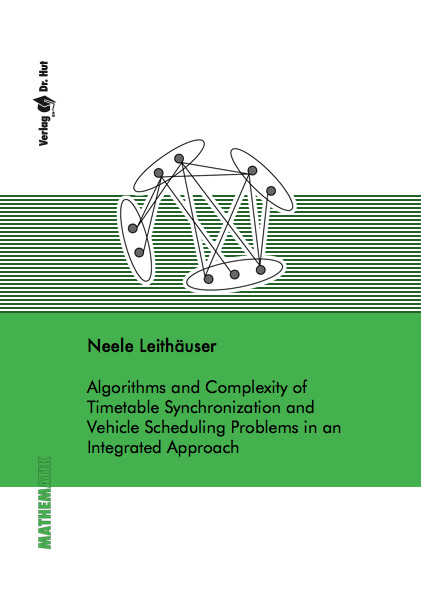Datenbestand vom 20. August 2019aktualisiert am 20. August 2019

# ISBN 9783843908726Euro 96,00 inkl. 7% MwSt

978-3-8439-0872-6, Reihe Mathematik

Neele Leithäuser
Algorithms and Complexity of Timetable Synchronization and Vehicle Scheduling Problems in an Integrated Approach

334 Seiten, Dissertation Technische Universität Kaiserslautern (2012), Hardcover, A5

## Zusammenfassung / Abstract

In this thesis, we discuss different models and algorithms that consider the problem of transfer quality optimization and vehicle scheduling in parallel on real world instances. We further extract the combinatorial subproblems MTQP, MTSP, and VSPVT that can be used to solve timetable synchronization and vehicle scheduling problems. We give an in-depth complexity analysis for them.

MTQP: Given a k-partite undirected network with edge weights, we seek to select one node per partition such that the total weight of all edges with both endpoints selected is maximal. We analyze the complexity of this problem and present approximation guarantees for the general and further restricted problems.

MTSP: Given a system of weighted equations of two variables of the form x-y=z, we seek to find a variable assignment that maximizes the total weight of all satisfied equations. We analyze the complexity of this problem and present approximation guarantees. We extend our results for variables restricted on certain domains.

VSPVT: Given a directed weighted k-partite flow network, we seek to select one node per partition such that the cost of a minimum cost flow in the resulting network of all arcs with both endpoints is minimal. We analyze the complexity of this problem and present approximation guarantees.